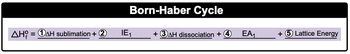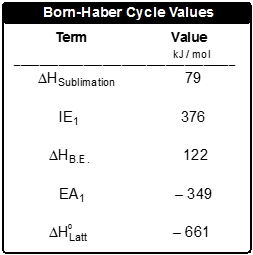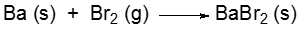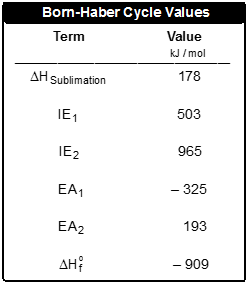## General Chemistry

Learn the toughest concepts covered in Chemistry with step-by-step video tutorials and practice problems by world-class tutors

11. Bonding & Molecular Structure

# Born Haber Cycle

In the Born-Haber Cycle, ionic solids are created through the ionization and eventual combination of elements.

The Born-Haber Cycle
1
concept

## Born Haber Cycle3m
Play a video:
Now, the born Haber cycle is a reaction outline that gives the steps for the formation of an ionic compound from the standard states of its elements. So I know that's a mouthful. The born Haber cycle is an extensive process. We're gonna go piece by piece, step by step to figure it out. Now recall first. Ah, formation equation shows the standard states of elements combining toe form one mole of product. Now remember, associated with the formation equation is our envelop, or heat of formation, which is Delta H F here. Standard heat of formation has that little circle there. It's telling me here that the formation of one mole of sodium chloride is accomplished by combining one mole of sodium solid with half a mole of chlorine gas. Remember information equations. We can have fractions and decimals as coefficients. Now, in order to calculate the entropy of formation, both elements must first be converted into their ionic gaseous forms. So we're starting out here with sodium solid. We're starting out here with CL to gas. What we need to do first is realized that we need to get sodium to its ionic form as a gas and we need to get chlorine to its ionic form as a gas as well. To do that, I first have to convert sodium solid into a gas. Going from a solid to a gas is called sublimation, so we're dealing with M. Papi of sublimation. Once I have it in its gas estate, I need to change it from a neutral metal to a positively charged metal. That means I'm removing electrons. Removing electrons has to do with ionization energy. And since I'm only removing one electron, it's ionization energy. One. If I needed to remove more than one electron, then I'd have to add an organization to organization three and so on. Remember, we talked about this under successive ionization energies. Next here, I need to separate CL two into two separate Clarence, so I have to break the bond. This is called entropy of dissociation here. Once we break the bond between the two chlorine, I just have one chlorine now as a gas, and then I have tow. Add an electron to it. Adding an electron is electron affinity, and since I'm only adding one electron, it's e a one. If I had to add more than one electron there will B e a to e a three and so on. Now that I have both of them in their ionic forms, they're gonna combine together to give me my solid remember, combining gashes ions to form a solid ionic product. This is connected to lattice energy, so it's a whole process. We have to do all of this just to get the entropy of formation. So I need to do Step one, which is entropy of sublimation. Step two would be ionization energy, which could be in more than one step. Step three. I'd have to do entropy of dissociation toe break a bond so I could get a single chlorine. Then I need to make it. Negatives would have to do electron affinity, which also could be more than one step. Once I get them into their ionic gas form, then I have lattice energy to complete it so he could say that and tilapia formation is composed of these five fundamental steps. So, like I told you, the born Haber cycle has a lot of parts to it. But just remember the method we used here to justify how sodium combines with chlorine to give us sodium chloride2
example

## Born Haber Cycle Example 11m
Play a video:
here. It says when setting up the steps of the born Haber cycle for potassium oxide. How maney ionization energies e. And how many electron affinities E A do you need? Remember, we have to get them into their ionic forms, right? And we need to potassium ions as gas to combine with one oxide ion as a gas. Then they will combine to give me potassium oxide solid realize here that we need oxygen to get a to minus charge. So we're not adding one electron. We're adding two electrons. That means that we're gonna need to do to electron affinities. So we need to electron affinities, which means this is out. This is out and this is out. And then finally, what else do we need? Well, we have one potassium solid initially, but at the end, we're gonna need to potassium. So we're gonna have toe do ionization energy twice. One for each of the potassium solids, and then both of them within undergo groups will have them as gas is actually. And then each one will have its electron plucked off. So we need to do to ionization energies one for each potassium as a neutral gas. So we need to do to electron affinities and to ionization energies, giving us e as our final answer.
3
Problem

Using the Born-Haber Cycle, demonstrate the formation of cesium chloride, CsCl, and calculate its enthalpy of formation.4
Problem

Calculate the lattice energy for the following formation equation: